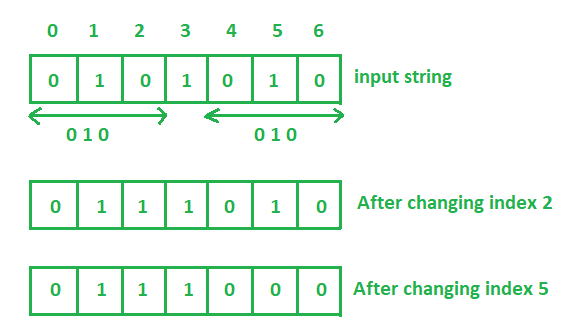# Minimum steps to remove substring 010 from a binary string

Given a binary string, the task is to count the minimum steps to remove substring “010” from this binary string.

Examples:

Input: binary_string = “0101010”
Output: 2
Switching 0 to 1 at index 2 and index 4 will remove the substring 010.
Hence the number of steps needed is 2.Input: binary_string = “010”
Output: 1
Switching any one 0 to 1 or 1 to 0 will remove the substring 010.
Hence the number of steps needed is 1.

## Recommended: Please try your approach on {IDE} first, before moving on to the solution.

Approach:

1. Iterate the string from beginning to end-2 of the binary string.
2. If in binary string continuously three characters are ‘0’, ‘1’, ‘0’ then any one character can be change so that one step will be count.
3. Increase the loop counter by 2.

Below is the implementation of the above approach:

## C++

 `// CPP program to calculate steps ` `// to remove substring 010 ` `// from a binary string ` ` `  `#include ` `using` `namespace` `std; ` ` `  `// Function to find the minimum steps ` `int` `minSteps(string str) ` `{ ` ` `  `    ``int` `count = 0; ` ` `  `    ``for` `(``int` `i = 0; i < str.length() - 2; i++) { ` ` `  `        ``if` `(str[i] == ``'0'``) { ` `            ``if` `(str[i + 1] == ``'1'``) { ` `                ``if` `(str[i + 2] == ``'0'``) { ` ` `  `                    ``// substring "010" found ` `                    ``count++; ` `                    ``i += 2; ` `                ``} ` `            ``} ` `        ``} ` `    ``} ` ` `  `    ``return` `count; ` `} ` ` `  `// Driver code ` `int` `main() ` `{ ` ` `  `    ``// Get the binary string ` `    ``string str = ``"0101010"``; ` ` `  `    ``// Find the minimum steps ` `    ``cout << minSteps(str); ` ` `  `    ``return` `0; ` `} `

## Java

 `// Java program to calculate steps ` `// to remove substring 010 ` `// from a binary string ` `import` `java.util.*; ` ` `  `class` `GFG{ ` ` `  `// Function to find the minimum steps ` `static` `int` `minSteps(String str) ` `{ ` ` `  `    ``int` `count = ``0``; ` ` `  `    ``for` `(``int` `i = ``0``; i < str.length() - ``2``; i++) { ` ` `  `        ``if` `(((``int``)str.charAt(i)) == ``'0'``) { ` `            ``if` `(str.charAt(i + ``1``) == ``'1'``) { ` `                ``if` `(str.charAt(i + ``2``) == ``'0'``) { ` ` `  `                    ``// substring "010" found ` `                    ``count++; ` `                    ``i += ``2``; ` `                ``} ` `            ``} ` `        ``} ` `    ``} ` ` `  `    ``return` `count; ` `} ` ` `  `// Driver code ` `public` `static` `void` `main(String args[]) ` `{ ` ` `  `    ``// Get the binary string ` `    ``String str = ``"0101010"``; ` ` `  `    ``// Find the minimum steps ` `    ``System.out.println(minSteps(str)); ` `} ` `} `

## Python3

 `# Python3 program to calculate steps ` `# to remove substring 010 ` `# from a binary string ` ` `  `# Function to find the minimum steps ` `def` `minSteps(``str``): ` `     `  `    ``count ``=` `0` `    ``i ``=` `0` `    ``while` `i < ``len``(``str``) ``-` `2``: ` `        ``if` `str``[i] ``=``=` `'0'``: ` `            ``if``(``str``[i ``+` `1``] ``=``=` `'1'``): ` `                ``if``(``str``[i ``+` `2``] ``=``=` `'0'``): ` `                     `  `                    ``# substring "010" found ` `                    ``count ``=` `count ``+` `1` `                    ``i ``=` `i ``+` `2` `        ``i ``=` `i ``+` `1` `     `  `    ``return` `count ` ` `  `# Driver code ` ` `  `# Get the binary string ` `str` `=` `"0101010"` ` `  `# Find the minimum steps ` `print``(minSteps(``str``)) ` `         `  `# This code is contributed  ` `# by Shashank_Sharma `

## C#

 `// C# program to calculate steps ` `// to remove substring 010 ` `// from a binary string ` `using` `System; ` ` `  `class` `GFG ` `{ ` ` `  `// Function to find the minimum steps ` `static` `int` `minSteps(``string` `str) ` `{ ` `    ``int` `count = 0; ` ` `  `    ``for` `(``int` `i = 0; i < str.Length - 2; i++)  ` `    ``{ ` ` `  `        ``if` `(((``int``)str[i]) == ``'0'``)  ` `        ``{ ` `            ``if` `(str[i + 1] == ``'1'``) ` `            ``{ ` `                ``if` `(str[i + 2] == ``'0'``) ` `                ``{ ` ` `  `                    ``// substring "010" found ` `                    ``count++; ` `                    ``i += 2; ` `                ``} ` `            ``} ` `        ``} ` `    ``} ` ` `  `    ``return` `count; ` `} ` ` `  `// Driver code ` `public` `static` `void` `Main() ` `{ ` ` `  `    ``// Get the binary string ` `    ``string` `str = ``"0101010"``; ` ` `  `    ``// Find the minimum steps ` `    ``Console.Write(minSteps(str)); ` `} ` `} ` ` `  `// This code is contributed by ChitraNayal `

## PHP

 ` `

Output:

```2
```

My Personal Notes arrow_drop_upCheck out this Author's contributed articles.

If you like GeeksforGeeks and would like to contribute, you can also write an article using contribute.geeksforgeeks.org or mail your article to contribute@geeksforgeeks.org. See your article appearing on the GeeksforGeeks main page and help other Geeks.

Please Improve this article if you find anything incorrect by clicking on the "Improve Article" button below.

Article Tags :
Practice Tags :

1

Please write to us at contribute@geeksforgeeks.org to report any issue with the above content.